In high-speed circuits, transmission line effects tend to distort signals on path that are long compared to the wavelength of the signals propagating on the path. This is rather the case in modern high speed backplain buses of digital computers. Therefore we investigate these effects.

 Parameters of Transmission Lines

The equivalent circuit for a transmission line is shown in figure below: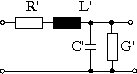Equivalent circuit of a transmission line

The parameters representing values for unit length are as follows: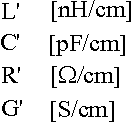Parameters of a transmission line

The charcteristic impedance of the transmission line is calculated as follows: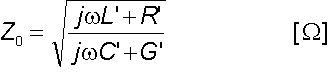Characteristic impedance of a transmission line

Since the losses of the transmission lines in digital system buses can be neglected, the characteristic impedance becomes real and has the following value: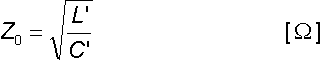Simplified charasteristic impedance

Another important parameter of a transmission line is the propagation time between the two ends. For long buses it is more dominant than the propagation delays caused by the line drivers. The propagation delay of a transmission line is calculated as follows: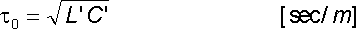Propagation delay on a transmission line

Finally, important calculated parameters of a transmission line are the reflection coefficients:Reflection coefficients of a transmission line

where RG and RL are the generator and load resistances, respectively.

 Phenomenons on Transmission Lines

Phenomenons on a transmission line is shown by the applet below. Adjustable parameters on it are: generator impedance ZG, characteristic impedance Z0 and load impedance ZL. In the case of the load impedance also infinite value can be input entering string inf. Finally X is for the location where the voltage is to be displayed. Its value must be between 0 and 100.

Impressing a voltage on the begin of the line it will not be present at the end of the line immediately. Instead it will propagate down to the end with a finite speed. It can be seen there only after the propagation delay.

Going into details, followings happen:

1. Impressing the voltage on the begin of the line it is divided on the two series impedances ZG and Z0. The voltage on impedance Z0 will travel as a voltage wave in the positive direction on the line.
2. When this wave reaches the load end it will be reflected in accordance with the load reflection coefficient rL. This wave will travel on the line in the negative direction.
3. When the wave propagating in the negative direction reaches the generator, a new reflexion occures in accordance with the generator reflection coefficient rG. This reflected wave will travel on the line in positive direction.
4. From this time point the whole phenomenon repeats from point 1.

The Applet

Observe the tranzient phenomenons for some typical parameter values!

• If the generator impedance is zero (0), and the load impedance is infinite (inf), reflection coefficients are 1 and -1. It causes the voltage on the load to undumped ringing between 0 és 2UG.
• If the generator impedance is zero (0), however the load impedance is not infinite but larger than the characteristic impedance of the line then the ringing on the load will be damped, although at the beginning we will experience some overshot.
• Next the generator impedance is still zero, however, the load impedance is finite and smaller than the characteristic impedance. In this case we will not experience overshot at the end of the line.
• If both the genarator and the load impedance are finite, there will be some ringing and the final voltage value on the load is the divided voltage between the two impedances ZG and ZL.
• The most important case: the load impedance is equal with the characteristic impedance of the line (matched end). In this case reflection coefficient of the load is zero, no reflection happens at the load end of the line.
• Another important case: impedance of the generator is equal with the characteristic impedance. In this case one reflection at the load end happens, however, at the generator end the negative wave is not reflected any more.
 Measuring the Line Impedance

Since it is diffucult to measure or calculate L and C of a transmission line the characteristic impedance is usually measured. The basis of the measurement ....

Web page design by Adam Traidman